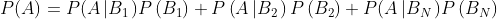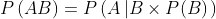Scan QR code or get instant email to install app

Question:

# Tony Borden, CFA, is analyzing the earnings of two companies. For each company, Borden estimates a probability that its earnings will exceed the consensus estimate. To estimate the probability that at least one of the companies will exceed its earnings estimate, Borden should use the:

1212The addition rule of probability is used to calculate the probability that at least one of two events will occur: P(A or B) = P(A) + P(B) − P(AB). The total probability rule is used to calculate the unconditional probability of an event given conditional probabilities related to the event:The multiplication rule of probability is used to calculate the joint probability that two events will occur together: